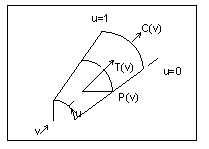Example: Fillet Surface Data Format
You can find a fillet surface where a round or a fillet is placed on a curved edge, or on an edge with non-constant arc radii. On a straight edge, you would use a cylinder to represent the fillet. The following illustration shows a fillet surface.Data format:
 pnt_spline P(v) spline running along the u = 0 boundary ctr_spline C(v) spline along the centers of the fillet arcs tan_spline T(v) spline of unit tangents to the axis of the fillet arcs
Parameterization:
R(v) = P(v) - C(v)

(x,y,z) = C(v) + R(v) * cos(u) + T(v) X R(v) * sin(u)